• 主要为大家详细介绍了OpenCV Python实现图像指定区域裁剪，文中示例代码介绍的非常详细，具有一定的参考价值，感兴趣的小伙伴们可以参考一下
• opencv3.4.3以后增加了专利，遇到pip install 无法安装时可以下载手都安装，亲测可用。 opencv-pythonopencv-contrib-python需要版本一致。
• 这个opencv包对应Python3.6版本，也是opencv_python包的最新版本。 opencv广泛用于计算机视觉开发，人脸检测和车牌号识别都是靠opencv算法的巨大支撑。
• opencv-python 3.4.1.15 opencv-contrib-python 3.4.1.15 win64版，opencv-python 3.4.1.15 opencv-contrib-python 3.4.1.15，支持版本：python3.4，3.5，3.6
• OpenCV python 图像坐标系 # -*- coding: utf-8 -*- import cv2 def main(): # 1.读取图片 打印图片形状 img_src = cv2.imread('./image/Lenna.png', cv2.IMREAD_UNCHANGED) img_copy = img_src.copy() print...
OpenCV python 图像坐标系
所需资源文件: 图片文件下载地址
# -*- coding: utf-8 -*-
import cv2

def main():

# 1.读取图片 打印图片形状
img_copy = img_src.copy()
print('Lenna 图片形状:', img_src.shape)

logo = cv2.resize(logo, (20, 20))
print('opencv_logo 图片形状:', logo.shape)

butterfly = cv2.resize(butterfly, (20, 20))
print('butterfly 图片形状:', butterfly.shape)

# 2.读取 坐标 y, x的值并打印
y = 100
x = 50
(b, g, r) = img_src[y, x]
print('蓝色:', b, '蓝色:', g, '红色:', r)

# 3.将 Logo butterfly 图片贴在原图上
img_src[100:100 + logo.shape, 300:300 + logo.shape] = logo[:, :, 0:3]  # 两张图片的shape不一样
img_src[300:300 + butterfly.shape, 100:100 + butterfly.shape] = butterfly[:, :, 0:3]

# 4.使用文字标识 坐标值
font = cv2.FONT_HERSHEY_SIMPLEX
cv2.putText(img_src, text='X=0,Y=0', org=(0, 0), fontFace=font, fontScale=0.5,
color=(0, 255, 0), thickness=2, bottomLeftOrigin=True)
cv2.putText(img_src, text='X=10,Y=30', org=(10, 30), fontFace=font, fontScale=0.5,
color=(0, 255, 0), thickness=2)
cv2.putText(img_src, text='X=100,Y=300', org=(100, 300), fontFace=font, fontScale=0.5,
color=(0, 255, 0), thickness=2)
cv2.putText(img_src, text='X=300,Y=100', org=(300, 100), fontFace=font, fontScale=0.5,
color=(0, 255, 0), thickness=2)

# 5.显示结果图片 移动图片位置
cv2.imshow('src', img_copy)
cv2.moveWindow('src', 0, 0)

cv2.imshow('img+logo', img_src)
cv2.moveWindow('img+logo', x=img_src.shape, y=0)

# 6.写入图片
cv2.imwrite('img_logo.jpg', img_src)

cv2.waitKey(0)

if __name__ == '__main__':
main()


处理结果图片展开全文• opencv_python-3.4.3-cp36-cp36m-win_amd64.whl opencv_python-3.4.3-cp37-cp37m-win_amd64.whl 64位，cp36和cp37两种
• 目录: 读取图像 显示图像 加载图像 从相机中读取视频 从文件播放视频 保存视频 图像灰度处理 图像二值化 划线 画矩形 画椭圆 ..........
• https://stackoverflow.com/questions/50854235/how-to-draw-chinese-text-on-the-image-using-cv2-puttextcorrectly-pythonopen
https://stackoverflow.com/questions/50854235/how-to-draw-chinese-text-on-the-image-using-cv2-puttextcorrectly-pythonopen
展开全文• PythonOpenCv的项目实践代码，供小伙伴们下载参考。
• OpenCV python 获取轮廓面积 处理图片[source.jpg] import cv2 import numpy as np def main(): # 1.导入图片 img_src = cv2.imread("source.jpg") # 2.灰度化与二值化 img_gray = cv2.cvtColor(img_src, ...
OpenCV python 获取轮廓面积
处理图片[source.jpg]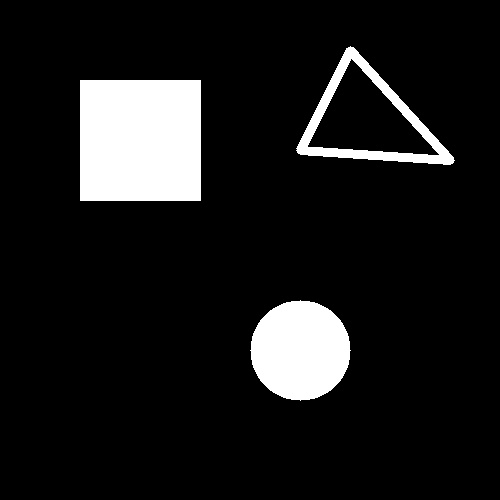import cv2
import numpy as np

def main():

# 1.导入图片

# 2.灰度化与二值化
img_gray = cv2.cvtColor(img_src, cv2.COLOR_BGR2GRAY)
ret, img_bin = cv2.threshold(img_gray, 127, 255, cv2.THRESH_BINARY)

# 3.连通域分析
img_contour, contours, hierarchy = cv2.findContours(img_bin, cv2.RETR_LIST, cv2.CHAIN_APPROX_SIMPLE)

# 4.轮廓面积打印
img_contours = []
for i in range(len(contours)):

area = cv2.contourArea(contours[i])
print("轮廓 %d 的面积是:%d" % (i, area))

img_temp = np.zeros(img_src.shape, np.uint8)
img_contours.append(img_temp)

cv2.drawContours(img_contours[i], contours, i, (255, 255, 255), -1)
cv2.imshow("%d" % i, img_contours[i])

# 5.显示结果
cv2.imshow("img_bin", img_bin)
cv2.imshow("img_src", img_src)
cv2.waitKey()
cv2.destroyAllWindows()

if __name__ == '__main__':
main()


处理结果：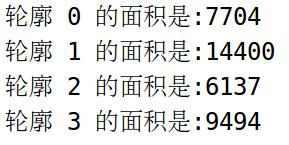void cv::findContours	(
InputArray 	image,  					//输入的处理图片;
OutputArrayOfArrays 	contours,		//输出轮廓
OutputArray 	hierarchy,				//输出轮廓的包含关系
int 	mode,							//寻找轮廓模式
int 	method,							//寻找 近似的方法
Point 	offset = Point() 				//所有轮廓坐标 增加偏移量
)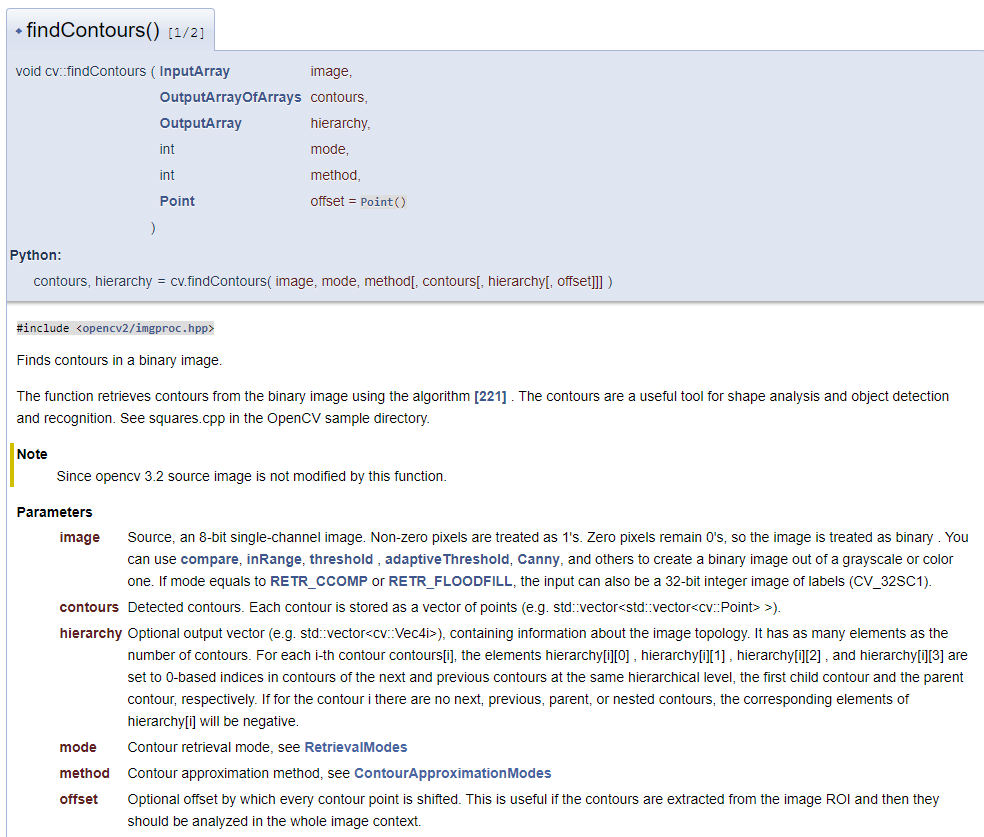# 只寻找 【外部区域】连通域 子连通域忽略。
contours, hierarchy = cv2.findContours(img_bin, cv2.RETR_EXTERNAL, cv2.CHAIN_APPROX_SIMPLE)

展开全文• opencv_python官方中文教程源码，其中段力辉老师文章中出现的许多代码不能运行，已经进行修改，敬请参考。其中段力辉老师的电子版也已经上传，欢迎下载
• 数十个编程实例让你从python-opencv的新手成长为入门汉。1天学习3个编程案例，10天即可以掌握如何用python实现图像处理。从最简单的画圆，画矩形，画复杂图形，到图像的预处理，图像的形态学处理，图像的特征提取等...
• OpenCV 3 Python3 图像自设 直接使用 只需修改文件路径即可
• ## opencvpython 图像去噪

万次阅读 2019-02-26 10:06:42
opencv python 图像去噪 Image Denoising  我们看到很多图像平滑技术如高斯模糊，Median模糊等，它们在移除数量小的噪音时在某种程度上比较好用。在这些技术里，我们取像素周围的一小部分邻居，做一些类似于高斯...
                           opencv python 图像去噪
Image Denoising
我们看到很多图像平滑技术如高斯模糊，Median模糊等，它们在移除数量小的噪音时在某种程度上比较好用。在这些技术里，我们取像素周围的一小部分邻居，做一些类似于高斯平均权重，中值等替换掉中间的元素。简单说，移除一个像素的噪音是基于本地邻居的。
噪音有一个属性，噪音一般被认为是具有零平均值的随机变量。假设一个像素噪音，p = p0 + n, 其中p0是像素的真实值，n是那个像素的噪音。你可以从不同图像取大量的同一个像素(N)并计算他们的平均值，理想情况下，你应该得到p=p0，因为均值是0.
OpenCV提供了这种技术的四种变体。
cv2.fastNlMeansDenoising（） - 使用单个灰度图像cv2.fastNlMeansDenoisingColored（） - 使用彩色图像。cv2.fastNlMeansDenoisingMulti（） - 用于在短时间内捕获的图像序列（灰度图像）cv2.fastNlMeansDenoisingColoredMulti（） - 与上面相同，但用于彩色图像。
Common arguments:
h：参数决定滤波器强度。较高的h值可以更好地消除噪声，但也会删除图像的细节 (10 is ok)hForColorComponents：与h相同，但仅适用于彩色图像。 （通常与h相同）templateWindowSize：应该是奇数。 （recommended 7）searchWindowSize：应该是奇数。 （recommended 21）
cv2.fastNlMeansDenoisingColored() 如上所述，它用于从彩色图像中去除噪声。 （噪音预计是高斯噪音）
import numpy as np
import cv2
import matplotlib.pyplot as plt

dst = cv2.fastNlMeansDenoisingColored(img,None,10,10,7,21)

plt.subplot(121),plt.imshow(img)
plt.subplot(122),plt.imshow(dst)
plt.show()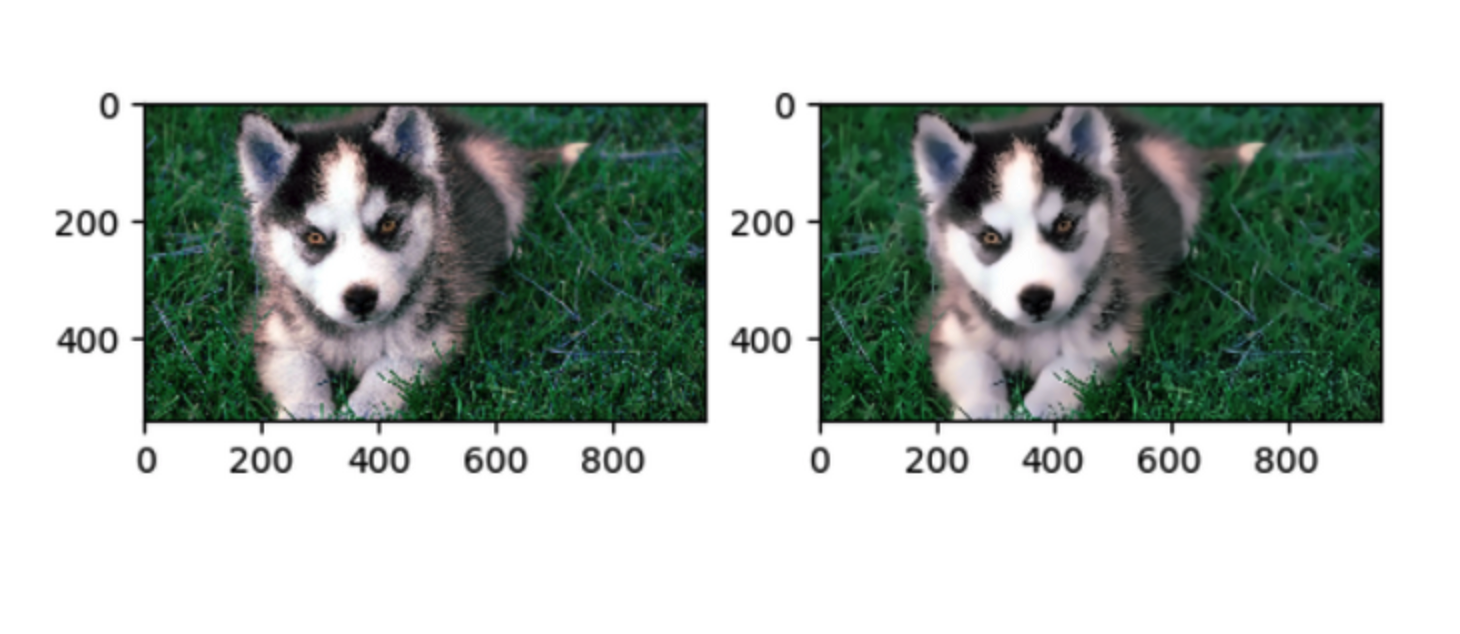cv2.fastNlMeansDenoisingMulti（）
现在我们将相同的方法应用于视频。 第一个参数是嘈杂帧的列表。 第二个参数imgToDenoiseIndex指定我们需要去噪的帧，因为我们在输入列表中传递了frame的索引。 第三个是temporalWindowSize，它指定了用于去噪的附近帧的数量。 在这种情况下，使用总共temporalWindowSize帧，其中中心帧是要去噪的帧。 例如，传递了5个帧的列表作为输入。 设imgToDenoiseIndex = 2和temporalWindowSize = 3.然后使用frame-1，frame-2和frame-3对帧-2进行去噪。
import numpy as np
import cv2
import matplotlib.pyplot as plt

cap = cv2.VideoCapture('test.mp4')

# create a list of first 5 frames
img = [cap.read() for i in range(5)]

# convert all to grayscale
gray = [cv2.cvtColor(i, cv2.COLOR_BGR2GRAY) for i in img]

# convert all to float64
gray = [np.float64(i) for i in gray]

# create a noise of variance 25
noise = np.random.randn(*gray.shape)*10

# Add this noise to images
noisy = [i+noise for i in gray]

# Convert back to uint8
noisy = [np.uint8(np.clip(i,0,255)) for i in noisy]

# Denoise 3rd frame considering all the 5 frames
dst = cv2.fastNlMeansDenoisingMulti(noisy, 2, 5, None, 4, 7, 35)

plt.subplot(131),plt.imshow(gray,'gray')
plt.subplot(132),plt.imshow(noisy,'gray')
plt.subplot(133),plt.imshow(dst,'gray')
plt.show()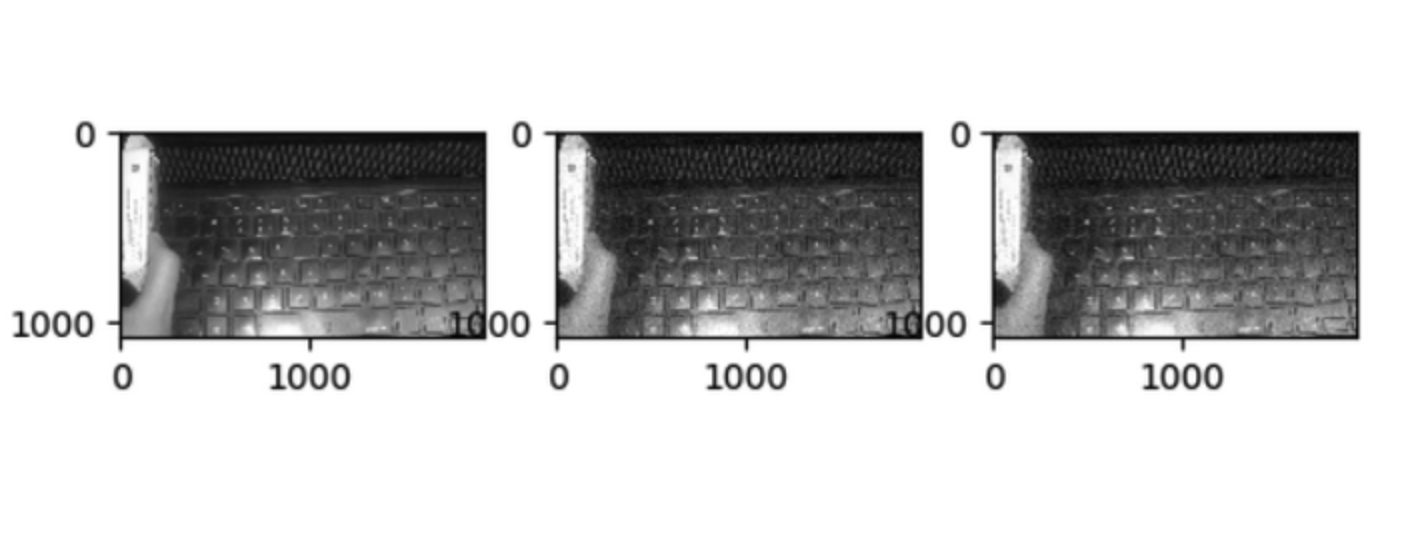【转载】：https://segmentfault.com/a/1190000015846441
https://zhuanlan.zhihu.com/p/40325840
展开全文• 支持向量机（support vector machines, SVM）是一种二分类模型，它的基本模型是定义在特征空间上的间隔最大的线性分类器，本文是 OpenCV的简单SVM例程
• 今天小编就为大家分享一篇python opencv 实现对图像边缘扩充，具有很好的参考价值，希望对大家有所帮助。一起跟随小编过来看看吧
• OpenCV官方教程中文版（For Python），OpenCV 3.0, Python 2.7。
• python 版本opencv 清晰，pdf,包括图像处理，识别等常用方法
• opencv for Python官方文档中文版！
• OpenCV-Python-Tutorial-中文版，是opencv官方文档的翻译版本。
• OpenCV 3计算机视觉 Python语言实现（第二版）（含示例代码）
• 主要为大家详细介绍了python+opencv实现动态物体的追踪，具有一定的参考价值，感兴趣的小伙伴们可以参考一下
• 用于Python的非官方的预构建的仅CPU的OpenCV软件包。 如果您希望从源代码编译绑定以启用其他模块（例如CUDA），请查看手动构建部分。 安装及使用 如果您已经安装了OpenCV先前/其他手动安装版本（=未通过pip进行...
• 主要为大家详细介绍了python利用opencv实现SIFT特征提取与匹配，文中示例代码介绍的非常详细，具有一定的参考价值，感兴趣的小伙伴们可以参考一下
• 两本python_opencv中文教程，OpenCV-Python-Toturial-中文版，OpenCV入门教程
• ## OpenCVPython 4.0安装与开发注意事项

千次阅读 多人点赞 2019-01-21 09:20:43
OpenCV4.0发布已经快两个月啦，Python社区终于推出了OpenCV Python4.0的安装包与扩展模块安装包，下载地址如下： https://pypi.org/project/opencv-python/ 安装OpenCV-Python 如果你是第一次使用OpenCV Python......

# opencvpythonpython 订阅